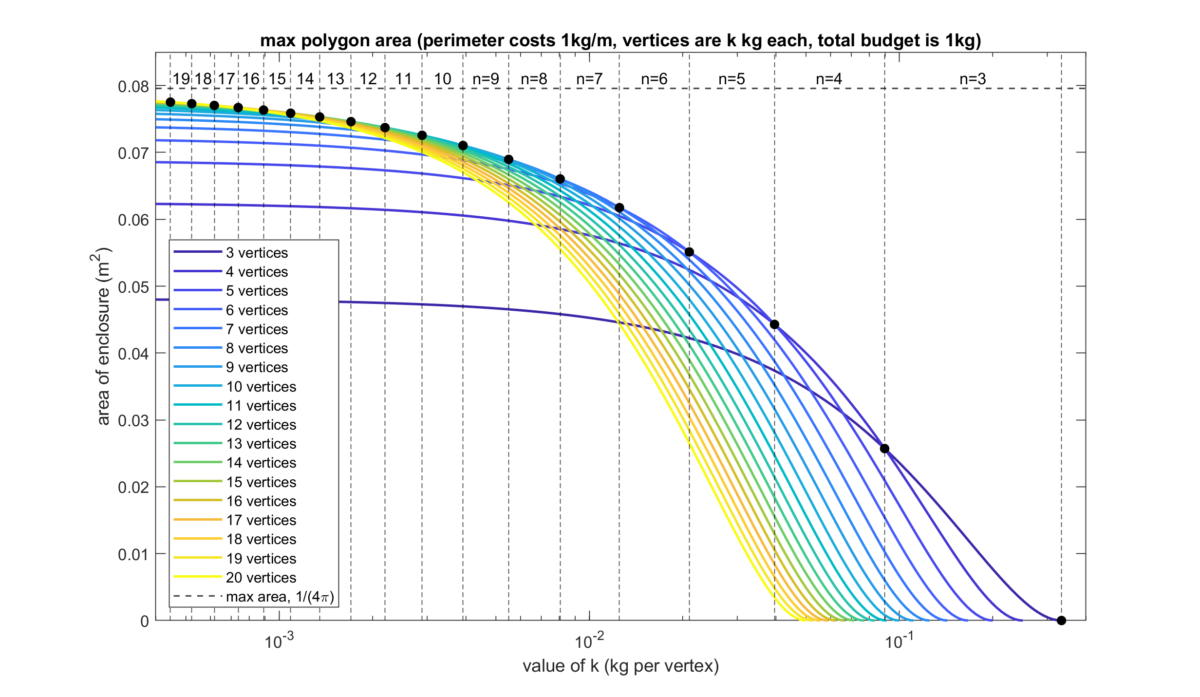# Polygons with perimeter and vertex budgetshis week’s Riddler Classic involves designing maximum-area polygons with a fixed budget on the length of the perimeter and the number of vertices. The original problem involved designing enclosures for hamsters, but I have paraphrased the problem to make it more concise.

You want to build a polygonal enclosure consisting of posts connected by walls. Each post weighs $k$ kg. The walls weigh $1$ kg per meter. You are allowed a maximum budget of $1$ kg for the posts and walls.

What’s the greatest value of $k$ for which you should use four posts rather than three?

Extra credit: For which values of $k$ should you use five posts, six posts, seven posts, and so on?

Here is my solution:
[Show Solution]